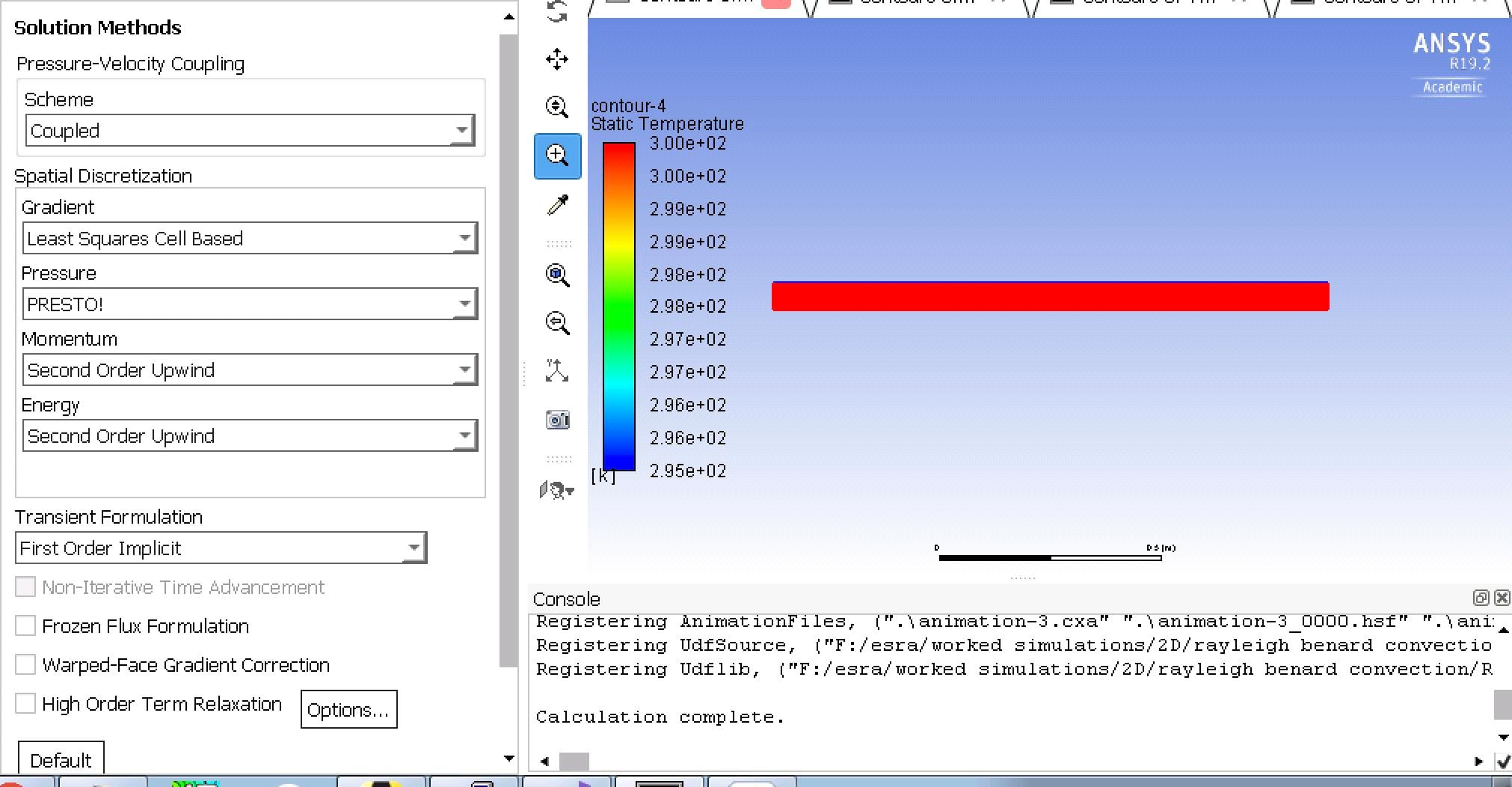## Fluids

•ehkh
Subscriber
Hi I am simulating a simple natural convection problem in a 2D rectangular cavity with the following boundary conditions: adiabatic top layer, periodic side walls and rigid non-slip bottom with random heat flux boundary condition applied over the faces of the layer using a UDF following this equation: Q= k*T*(-exp(-h)+ epsilon*[rand(x)-0.5])/h , where rand is a one dimensional array of random numbers uniformly distributed in the range of [0,1], k,T and h are the thermal conductivity, fluid temperature and vertical hight of the cavity respectively.

When using the UDF as heat flux boundary condition at the bottom layer, I supposed to see plumes or vortex on the temperature contour profile. I am expecting to see something similar to previous work done using SNS code, please refer to Figure 6 (Attached here) in the Journal article (Hattori et. at, 2015) https://doi.org/10.1016/j.ijheatmass...er.2015.03.060

please note that I am running a transient simulation where time step size equals to 0.0001 and Ra equals to 10^9 .
Please advice me with the correct setup for the heat flux boundary condition.
Many thanks.

•DrAmine
Ansys Employee

What is now the problem? Why are you creating a new thread?

•ehkh
Subscriber

Hi,

I am posting this to seek help in in running this simulation as I didn't get the expected results.

As I mentioned above, when using the UDF as heat flux boundary condition at the bottom layer, I am expecting to see vortex on the temperature contour profile, similar to previous work done using SNS code, please refer to the Figure attached above from a journal article. BUT the problem is that I get nothing, the water in the domain is not heating up either, however I have checked that the values of heat flux returned by my UDF is correct and matching the values used by the author of the above journal.

What I am doing at this stage as a PhD student is to build a model in Fluent of a natural convection problem previously solved using SNS code and compare the results.

Esra'a

•Rob
Ansys Employee

Is the wall directly on the fluid region, and if you plot temperature without the contour node values what do you see? I assume you're using a temperature dependent density?

•ehkh
Subscriber

Yes, the wall is directly on fluid region (water, Pr=7 and Ra=10^9).

Do you mean I need to use density based solver instead of pressure based solver? I used pressure based solver since I am simulating incompressible flow?

Also, whats the proper methods of discretisation should I use? any advices please?

I used the following methods in my model, with a time step size of 0.0001, please let me know if I need to modify them.A plot of temperature without the contour node values presented in the GUI above at time step =  414.

•Rob
Ansys Employee

No, pressure based solver is correct for this type of application. What are your material properties?  You do have a temperature range, so that part is working. Time step is based on the flow speed and cell size.

•DrAmine
Ansys Employee

Are you using temperature dependent density?

•ehkh
Subscriber

What do you mean by temperature dependant density? If I understand your question, I am using Boussinesq approximation with a constant thermal expansion coefficient.

I have tried to apply a temperature different of 5 degrees between top and bottom layer, with some perturbation at the bottom.  I have also tried to apply random heat flux at the bottom layer, ranging between 220-214 Watt/m2 . BUT it seems that the water in the domain heated up to the temp of the bottom layer!! I could not see any plumes or vortex.

•DrAmine
Ansys Employee

Okay you are using that Boussinesq fudge which is okay for small temeprture differences or say delta_T*ThermalExpansion<<1.

And gravity is on? And you are trying top reproduce a paper? Which models are used in the paper? Is it a CFD or exp. paper? As you know we are not a library having all papers in the mind that is help us to understand to be able to help you.

•ehkh
Subscriber

Yes, I turned gravity on, and turned the energy equation on too.

To validate my model, I am trying to perform a simulation using the same geometry, grid, boundary conditions and setups of previous work that used the SNS code as a numerical method, not an exp. paper.

•ehkh
Subscriber

It is good to mention that I used the same setup to simulate steady laminar simulation, with delta t equals 20 degrees and lower rayleigh number, and Rayleigh Benard cells appears successfully after convergence. Now I am aiming to simulate in this problem is a transient simulation with very fine grid (DNS) in Fluent.

•DrAmine
Ansys Employee

We do not claim having DNS capabilities in Fluent. DNS is very expensive. It scales with Re^11/4 and require high order numerics (beyound CDS).

You can mimic that with Laminar or better with LES with Cs=0.0 but you need to resolve everything.

•Rob
Ansys Employee

If you have an infinitely fine mesh and small enough time step you're sort of there: not with a student licence through!

•ehkh
Subscriber

At this step I am running RANs and LES, for this simulation I am using a grid size of 0.0025 which gives 200 and 4000 cells the vertical and on the horizontal walls respectively. I might go for finer once I make sure that I get a reasonable results.

I am using ANSYS on a university super machine with a full software licence.

•DrAmine
Ansys Employee

You need to check if the spatial and temporal resolution are enough for LES. You need at least to resolve 80% of the turbulence spectrum to talk about a good LES. This means at last 5 cells across the integral length scale. As you start from quiescent run the scales are at the beginning very small but need to be resolved 2.

Use LES with Wale.

Near the wall the resolution here is very very important as it triggers the whole plume.

CFL lower than 0.5 is must here.

Good Luck!

•DrAmine
Ansys Employee

and 3D!